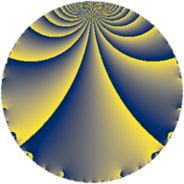# Properties

 Label 95.2.aLevel $95$ Weight $2$ Character orbit 95.a Rep. character $\chi_{95}(1,\cdot)$ Character field $\Q$ Dimension $7$ Newform subspaces $2$ Sturm bound $20$ Trace bound $1$

# Related objects

## Defining parameters

 Level: $$N$$ $$=$$ $$95 = 5 \cdot 19$$ Weight: $$k$$ $$=$$ $$2$$ Character orbit: $$[\chi]$$ $$=$$ 95.a (trivial) Character field: $$\Q$$ Newform subspaces: $$2$$ Sturm bound: $$20$$ Trace bound: $$1$$ Distinguishing $$T_p$$: $$2$$

## Dimensions

The following table gives the dimensions of various subspaces of $$M_{2}(\Gamma_0(95))$$.

Total New Old
Modular forms 12 7 5
Cusp forms 9 7 2
Eisenstein series 3 0 3

The following table gives the dimensions of the cuspidal new subspaces with specified eigenvalues for the Atkin-Lehner operators and the Fricke involution.

$$5$$$$19$$FrickeDim
$$+$$$$-$$$-$$$4$$
$$-$$$$+$$$-$$$3$$
Plus space$$+$$$$0$$
Minus space$$-$$$$7$$

## Trace form

 $$7 q - q^{2} + 4 q^{3} + 9 q^{4} - q^{5} - 4 q^{6} + 4 q^{7} - 9 q^{8} + 11 q^{9} + O(q^{10})$$ $$7 q - q^{2} + 4 q^{3} + 9 q^{4} - q^{5} - 4 q^{6} + 4 q^{7} - 9 q^{8} + 11 q^{9} + 3 q^{10} - 4 q^{11} - 4 q^{12} + 10 q^{13} - 12 q^{14} + q^{16} + 6 q^{17} - 29 q^{18} + q^{19} - 7 q^{20} - 16 q^{21} - 12 q^{22} - 12 q^{23} - 28 q^{24} + 7 q^{25} - 2 q^{26} + 4 q^{27} + 4 q^{28} - 6 q^{29} - 4 q^{30} + 8 q^{31} - 9 q^{32} + 12 q^{33} + 14 q^{34} - 4 q^{35} + 29 q^{36} + 14 q^{37} - 3 q^{38} - 8 q^{39} + 15 q^{40} + 14 q^{41} + 16 q^{42} + 12 q^{44} - 5 q^{45} + 12 q^{46} - 12 q^{47} + 48 q^{48} + 31 q^{49} - q^{50} - 32 q^{51} + 22 q^{52} + 6 q^{53} - 12 q^{55} + 4 q^{56} + 2 q^{58} - 20 q^{59} - 16 q^{60} + 18 q^{61} + 48 q^{62} - 12 q^{63} - 15 q^{64} + 6 q^{65} - 8 q^{66} - 16 q^{67} + 2 q^{68} + 16 q^{69} + 4 q^{70} - 24 q^{71} - 65 q^{72} + 30 q^{73} + 30 q^{74} + 4 q^{75} + 7 q^{76} - 32 q^{77} - 16 q^{79} - 7 q^{80} + 15 q^{81} - 2 q^{82} - 32 q^{83} - 24 q^{84} - 2 q^{85} - 24 q^{86} - 64 q^{87} - 16 q^{88} + 6 q^{89} + 39 q^{90} - 16 q^{91} - 20 q^{92} - 56 q^{93} - 52 q^{94} - 7 q^{95} - 28 q^{96} + 50 q^{97} + 23 q^{98} - 20 q^{99} + O(q^{100})$$

## Decomposition of $$S_{2}^{\mathrm{new}}(\Gamma_0(95))$$ into newform subspaces

Label Dim $A$ Field CM Traces A-L signs $q$-expansion
$a_{2}$ $a_{3}$ $a_{5}$ $a_{7}$ 5 19
95.2.a.a $3$ $0.759$ 3.3.148.1 None $$1$$ $$2$$ $$3$$ $$0$$ $-$ $+$ $$q+\beta _{1}q^{2}+(1-\beta _{1}-\beta _{2})q^{3}+(\beta _{1}+\beta _{2})q^{4}+\cdots$$
95.2.a.b $4$ $0.759$ 4.4.11344.1 None $$-2$$ $$2$$ $$-4$$ $$4$$ $+$ $-$ $$q+\beta _{2}q^{2}-\beta _{3}q^{3}+(1+\beta _{1}-\beta _{2})q^{4}+\cdots$$

## Decomposition of $$S_{2}^{\mathrm{old}}(\Gamma_0(95))$$ into lower level spaces

$$S_{2}^{\mathrm{old}}(\Gamma_0(95)) \cong$$ $$S_{2}^{\mathrm{new}}(\Gamma_0(19))$$$$^{\oplus 2}$$